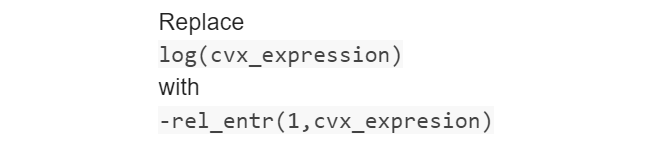# 这个问题怎么解决呀,希望大家帮忙看看

Disciplined convex programming error:
Illegal operation: {invalid} - {constant}

z = plus( x, y, true, cheat );

R_sub1=log2(1+A/u(ii,iter)) -B*(u1(ii)-u(ii,iter));

One of the input data on the right-hand-side is `NaN` or -Inf or Inf.

Input data being `NaN` can happen if you use the results of one problem as input data to another problem, but the first problem did not solve to otimality; hence the “optimal” values of its CVX variables are `NaN`.

Is `u(ii,iter)` the variable?
If `u` is variable, it can not be expressed by `log2(1+A/u(ii,iter)) `.With regard to `log()`, you can refer to this link— https://ask.cvxr.com/t/cvxquad-how-to-use-cvxquads-pade-approximant-instead-of-cvxs-unreliable-successive-approximation-for-gp-mode-log-exp-entr-rel-entr-kl-div-log-det-det-root

there are some expression which can’t be express by CVX directly.

And this link ---- Writing x*log(1+x/y)

1 Like